# Sector

The perimeter of a circular sector with an angle 1.8 rad is 64 cm.

Determine the radius of the circle from which the sector comes.

r =  35.56 cm

### Step-by-step explanation: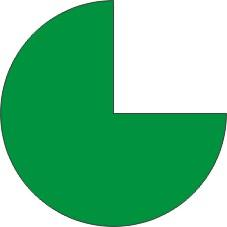Did you find an error or inaccuracy? Feel free to write us. Thank you!#### You need to know the following knowledge to solve this word math problem:

We encourage you to watch this tutorial video on this math problem:

## Related math problems and questions:

• Circle sectorThe circular sector with a central angle 160° has an area 452 cm2. Calculate its radius r.
• Circle and angleWhat is the length of the arc of a circle with radius r = 207 mm with cental angle 5.33 rad?
• Chord MNChord MN of circle has distance from the center circle S 120 cm. Angle MSN is 64°. Determine the radius of the circle.
• Circular sector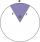I have a circular sector with a length 15 cm with an unknown central angle. It is inscribed by a circle with radius 5 cm. What is the central angle alpha in the circular sector?
• Arc-sectorarc length = 17 cm area of sector = 55 cm2 arc angle = ? the radius of the sector = ?
• Chord - TSThe radius of circle k measures 68 cm. Arc GH = 47 cm. What is TS?
• Quarter circleWhat is the radius of a circle inscribed in the quarter circle with a radius of 100 cm?
• Quadrant IICalculate the radius of the quadrant, which area is equal to area of circle with radius r = 15 cm.
• Round skirt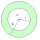The cut on the round skirt has the shape of an annulus. Determine how much m² of fabric will be consumed on an 80 cm long skirt. The circumference of the waist is a circle with a smaller radius and is 69 cm.
• Big cubeCalculate the surface of the cube, which is composed of 64 small cubes with an edge 1 cm long.
• Two annulusesThe area of the annular circle formed by two circles with a common center is 100 cm2. The radius of the outer circle is equal to twice the radius of the inner circle. Determine the outside circle radius in centimeters.
• Wheels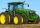Small tractor wheel with a diameter of 60 cm must be rotated 8 times to overcome some pathway. How many times must turn the big tractor wheel with a radius 60 cm to overcome the same distance?
• Arc and segmentCalculate the length of circular arc l, area of the circular arc S1 and area of circular segment S2. Radius of the circle is 11 and corresponding angle is (2)/(12) π.
• Arc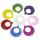What area of a circle occupied the flowers planted in the arc of a circle with radius 3 m with central angle 45°?
• Wire D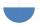Wire length 1 m is bent so that it forms a semicircle circuit (including the diameter). Determine the radius of the semicircle.
• Four circles1) Calculate the circle radius if its area is 400 cm square 2) Calculate the radius of the circle whose circumference is 400 cm. 3) Calculate circle circumference if its area is 400 cm square 4) Calculate the circle's area if perimeter 400 cm.
• Circle arcCircle segment has a circumference of 135.26 dm and 2096.58 dm2 area. Calculate the radius of the circle and size of the central angle.